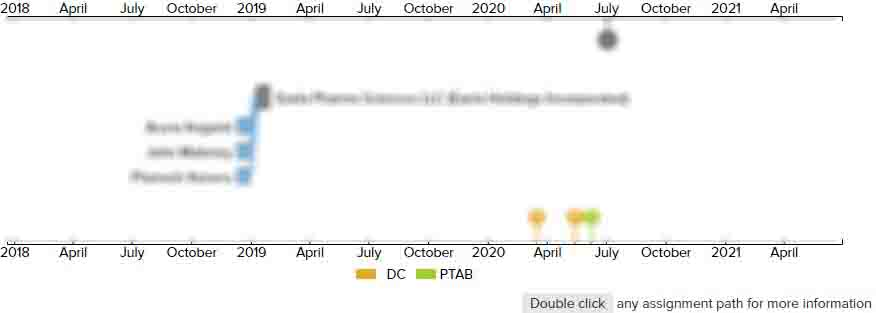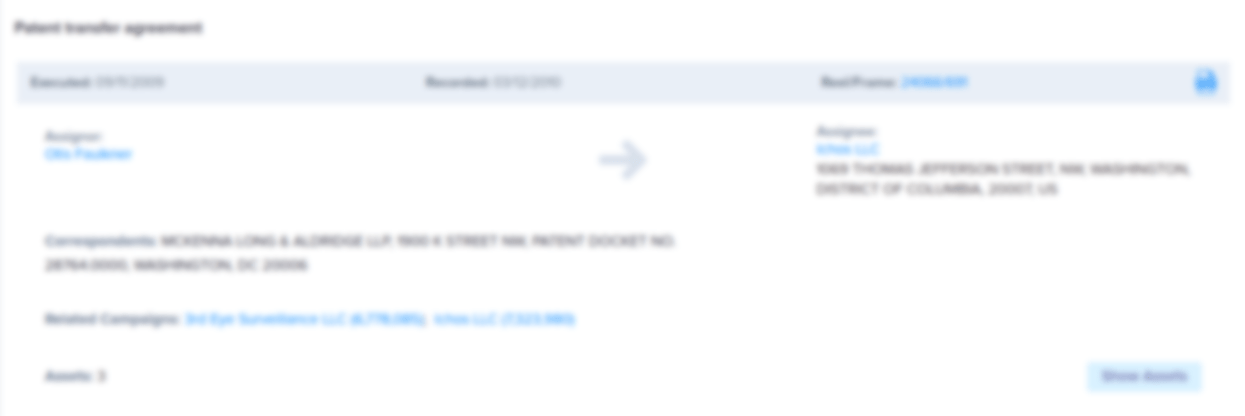# Sequencing data analysis method, device and computer-readable medium for microsatellite instability

• US 10,998,084 B2
• Filed: 06/06/2018
• Issued: 05/04/2021
• Est. Priority Date: 09/06/2017
• Status: Active Grant
##### First Claim
Patent Images

1. A method for analyzing sequencing data of microsatellite instability, comprising the following steps:

• S1;

performing Next Generation Sequencing (NGS) on a plurality of test samples and a plurality of normal samples to obtain sequencing data spanning Microsatellite Instability (MSI) locus to be determined in the plurality of test samples and the plurality of normal samples to identify a stable or unstable status of each MSI locus in each of the plurality of test samples and the plurality of normal samples to provide a genomic locus suitable for use as a microsatellite instability indicator;

S2;

for the sequencing data obtained in the step S1, using any one of the following three criteria for analysis, and if any of the three criteria is satisfied, then determining the MSI locus of a test sample is unstable;

S2-1;

according to the sequencing data obtained in the step S1, calculating a plurality of principal repeat unit species at the MSI locus for each of the plurality of test samples and each of the plurality of normal samples;

a tallying number (Ni) of the plurality of principal repeat unit species in the each of the plurality of normal samples, and calculating mean value [mean(Ni)] of the Ni and standard deviation [sd(Ni)];

if a number of the plurality of principal repeat unit species at the MSI locus in the test sample is larger than mean(Ni)+x*sd(Ni), then determining the MSI locus in the test sample is an unstable microsatellite locus, wherein x is a coefficient of standard deviation, and x=3;

S2-2;

according to the sequencing data obtained in the step S1, calculating the plurality of principal repeat unit species at the MSI locus of the each of the plurality of test samples and the each of the plurality of normal samples; and

if the plurality of principal repeat unit species that have not appeared in any of the plurality of normal samples are found in the test sample at the MSI locus, then determining the MSI locus in the test sample is the unstable microsatellite locus; and

S2-3;

according to the sequencing data obtained in the step S1, pooling all of the plurality of normal samples as a whole, calculating a plurality of population principal repeat unit species in all of the plurality of normal samples, and then calculating a proportion of the plurality of population principal repeat unit species in the each of the plurality of normal samples, performing statistical analysis according to the proportion to obtain a distribution reference set and calculate median [Q2(Ri)], first quartile [Q1(Ri)], and third quartile [Q3(Ri)] of the proportion, and calculating a proportion (RTi) of the plurality of population principal repeat unit species in the each of the plurality of test samples; and

if RTi>

Q2(Ri)+1.5*(Q3(Ri)−

Q1(Ri)) or RTi<

Q2(Ri)−

1.5*(Q3(Ri)−

Q1(Ri)), then determining that the MSI locus in the test sample is the unstable microsatellite locus.

• ##### 2 Assignments
Timeline View
Assignment View×
×